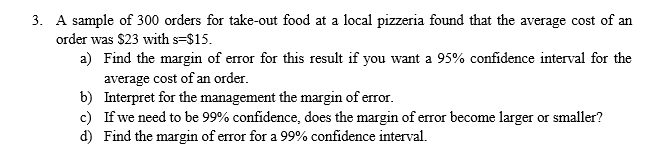# A sample of 300 orders for take-out food at a local pizzeria found that the average cost of anorder was \$23 with s-\$15.a) Find the margin of error for this result if you want a 95% confidence interval for theaverage cost of an order.Interpret for the management the margin of error.If we need to be 99% confidence, does the margin of error become larger or smaller?Find the margin of error for a 99% confidence interval.b)c)d)

Question
92 viewshelp_outlineImage TranscriptioncloseA sample of 300 orders for take-out food at a local pizzeria found that the average cost of an order was \$23 with s-\$15. a) Find the margin of error for this result if you want a 95% confidence interval for the average cost of an order. Interpret for the management the margin of error. If we need to be 99% confidence, does the margin of error become larger or smaller? Find the margin of error for a 99% confidence interval. b) c) d) fullscreen
check_circle

Step 1

Obtain the critical value:

The required confidence level is 100*(1–α)% = 95%.

The required conditions for using t distribution to estimate the confidence interval about mean is given below:

• The population standard deviation σ must be unknown.
• Either the sample size (n) must be greater than 30 or the population must be normally distributed.

Here, the sample size is n = 300 (which is greater than 30) and the population standard deviation is unknown.

The critical value is obtained as 1.968 from the calculation given below:

Step 2

a.

Find the margin of error of the confidence interval with 95% confidence level:

Given that, the sample standard deviation is s = \$15,

The sample mean is x-bar = \$23,

The sample size is n = 300,

The critical value is t(α/2, n–1) = t0.025,299 = 1.968.

The margin of error of the confidence interval with 95% confidence level is obtained as 1.7043 from the calculation given below:

Step 3

b.

Interpretation of margin of error:

The margin of error gives the accuracy of the estimate. It says the error for the statistic to be parameter.

Here, the margin of error is E = 1.7043.

From this it can be said that, we are 95% confident t...

### Want to see the full answer?

See Solution

#### Want to see this answer and more?

Solutions are written by subject experts who are available 24/7. Questions are typically answered within 1 hour.*

See Solution
*Response times may vary by subject and question.
Tagged in

### Other